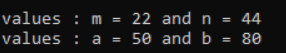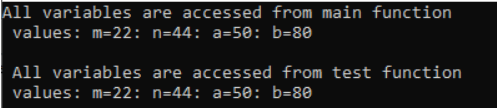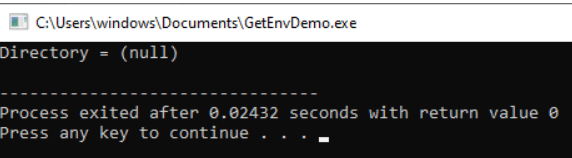# Types of Variables in C

## Types of Variables in C Language:

In this article, I am going to discuss the Types of Variables in C Language with Examples. Please read our previous article where we discussed the basics of C Language Variables. Based on the scope and lifetime of a variable, variables are categorized into three types. They are as follows

1. Local variable
2. Global variable
3. Environment variable

Note: The Scope tells about the visibility (i.e., from where this variable is visible), whereas the lifetime tells about the durability (i.e. how much time the value in the variable is valid).

##### Local Variables in C Language:

The variables declared inside a function are known as local variables in C. The scope of local variables in C will be within the function only i.e. we can’t access a local variable from outside the function in which it is declared. These variables are declared within the function and can’t be accessed outside the function.

The lifetime of a local variable is throughout the function i.e. memory to the local variables allocated when the function execution started and will become invalid once the function completes its execution.

##### Example to Understand Local Variables in C Language:

In the following example, m and n variables are having scope within the main function only. These are not visible to the test function. Likewise, a and b variables are having scope within the test function only. These are not visible to the main function.

```include <stdio.h>
void test ();
int main()
{
int m = 22, n = 44;		// m, n are local variables of main function
/*m and n variables are having scope within this main function only. These are not visible to test function.*/
/* If you try to access a and b in this function, you will get 'a' undeclared and 'b' undeclared error */
printf ("\n values : m = %d and n = %d", m, n);
test();
}

void test()
{
int a = 50, b = 80;		// a, b are local variables of test function
/* a and b variables are having scope within this test function only. These are not visible to main function.*/
/* If you try to access m and n in this function, you will get 'm' undeclared and 'n' undeclared error */
printf ("\n values : a = %d and b = %d", a, b);
}```

Output:##### Global Variables in C Language:

The variables which are declared outside the function are known as global variables in C. The scope of global variables will be throughout the program. These variables can be accessed from anywhere in the program.

The lifetime of a global variable is throughout the program, i.e. memory to the global variables will be allocated when the execution of the program is started and will become invalid after finishing the execution of the program.

##### Example to Understand Global Variables in C:

In the following example, the variables a, b, m, and n are declared outside the scope of any function. So, these variables are visible to the main function and all other sub-functions.

```#include<stdio.h>
void test();
int m = 22, n = 44;
int a = 50, b = 80;
int main()
{
printf("All variables are accessed from main function");
printf("\n values: m=%d: n=%d: a=%d: b=%d", m, n, a, b);
test();
}
void test()
{
printf("\n\n All variables are accessed from" \ " test function");
printf("\n values: m=%d: n=%d: a=%d: b=%d", m ,n, a, b);
}```
###### Output:##### Environment Variables in C Language:

The environment variable is a variable that will be available for all C applications and C programs. We can access these variables from anywhere in a C program without declaring and initializing in an application or C program.

The inbuilt functions which are used to access, modify, and set these environment variables are called environment functions. There are 3 functions that are used to access, modify, and assign an environment variable in C. They are,

1. setenv()
2. getenv()
3. putenv()

##### getenv() Function in C Language:

This function gets the current value of the environment variable. Let us assume that the environment variable DIR is assigned to “/usr/bin/test/”.

##### Example to understand getenv() Function in Language
```#include <stdio.h>
#include <stdlib.h>
int main() {
printf("Directory = %s\n", getenv("DIR"));
return 0;
}```
###### Output:##### setenv() Function in C Language:

This function sets the value for the environment variable. Let us assume that the environment variable “FILE” is to be assigned “/usr/bin/example.c”.

##### Example to understand setenv() Function in Language
```#include <stdio.h>
#include <stdlib.h>
int main()
{
setenv("FILE","/usr/bin/example.c",50);
printf("File = %s\n", getenv("FILE"));
return 0;
}```

Output: File = /usr/bin/example.c

##### putenv() Function in C Language:

This function modifies the value of the environment variable. The below example program shows how to modify an existing environment variable value.

##### Example to understand putenv() Function in Language
```#include <stdio.h>
#include <stdlib.h>
int main()
{
setenv("DIR","/usr/bin/example/",50);
printf("Directory name before modifying = " \ "%s\n", getenv("DIR"));

putenv("DIR=/usr/home/");
printf("Directory name after modifying = " \ "%s\n", getenv("DIR"));
return 0;
}```

Output:
Directory name before modifying = /usr/bin/example/
Directory name after modifying = /usr/home/

#### Interview Questions on Variables in C Programming Language:

##### Question 1: What will be the output of the below program
```#include <stdio.h>
int main()
{
printf("%d", number);
int number;
return 0;
}```

Output: Compilation Error. This is because the scope of the local variable starts from the point where we declared. In the above example, we are trying to access the variable before we are declaring it.

##### Question 2: What will be the output of the below program?
```#include <stdio.h>
int main()
{
int number = 10;
int number = 20;
printf("%d", number);
return 0;
}```

Output: Compilation Error. This is because Multiple declarations of the local variables with the same name and in the same scope are not allowed. In the above example, we are trying to declare a Local variable with the same name more than once

##### Question 3: What will be the output of the below program?
```#include <stdio.h>

int main()
{
printf("%d", number);
return 0;
}

int number = 10;```

Output: undeclared variable error. This is because the scope of the global variable also starts from the point where it is declared. In the above example, we are declaring the Global variable after we are using it in the “main” function.

##### Question 4: What will be the output of the below program?
```#include <stdio.h>

int number = 10;
number = 20;

int main()
{
printf("%d", number);
return 0;
}```

Output: re-definition error. This is because the Re-definition of Global variables is not allowed in the C language. In the above example, we are trying to redefine the Global variable with number= 20.

##### Question 4: What will be the output of the below program?
```#include <stdio.h>

int number1 = 10;
int number2 = number1 ;
int main()
{
printf("%d", number1);
return 0;
}```

Output: Compilation Error. This is because we cannot assign directly one “Global variable” to another “Global variable” outside the function. In the above example, we are trying to assign the Global variable number1 to another Global variable number2

In the next article, I am going to discuss Functions in C Language. Here, in this article, I try to explain the Types of Variables in C Language with examples and I hope you enjoy this Type of Variables in C Language with Examples article. I would like to have your feedback. Please post your feedback, question, or comments about these Types of Variables in the C Language article.